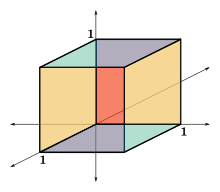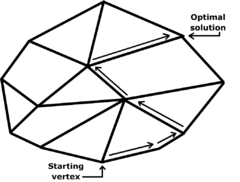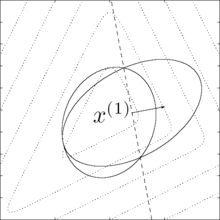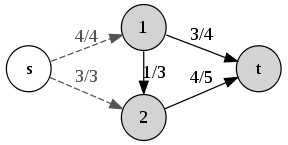# Criss-cross algorithm

﻿
Criss-cross algorithmThe criss-cross algorithm visits all 8 corners of the Klee–Minty cube in the worst case. It visits 3 additional corners on average. The Klee–Minty cube is a perturbation of the cube shown here.

In mathematical optimization, the criss-cross algorithm denotes a family of algorithms for linear programming. Variants of the criss-cross algorithm also solve more general problems with linear inequality constraints and nonlinear objective functions; there are criss-cross algorithms for linear-fractional programming problems, quadratic-programming problems, and linear complementarity problems.

Like the simplex algorithm of George B. Dantzig, the criss-cross algorithm is not a polynomial-time algorithm for linear programming. Both algorithms visit all 2D corners of a (perturbed) cube in dimension D, the Klee–Minty cube (after Victor Klee and George J. Minty), in the worst case. However, when it is started at a random corner, the criss-cross algorithm on average visits only D additional corners. Thus, for the three-dimensional cube, the algorithm visits all 8 corners in the worst case and exactly 3 additional corners on average.

## History

The criss-cross algorithm was published independently by Tamás Terlaky and by Zhe-Min Wang; related algorithms appeared in unpublished reports by other authors.

## Comparison with the simplex algorithm for linear optimizationIn its second phase, the simplex algorithm crawls along the edges of the polytope until it finally reaches an optimum vertex. The criss-cross algorithm considers bases that are not associated with vertices, so that some iterates can be in the interior of the feasible region, like interior-point algorithms; the criss-cross algorithm can also have infeasible iterates outside the feasible region.

In linear programming, the criss-cross algorithm pivots between a sequence of bases but differs from the simplex algorithm of George Dantzig. The simplex algorithm first finds a (primal-) feasible basis by solving a "phase-one problem"; in "phase two", the simplex algorithm pivots between a sequence of basic feasible solutions so that the objective function is non-decreasing with each pivot, terminating when with an optimal solution (also finally finding a "dual feasible" solution).

The criss-cross algorithm is simpler than the simplex algorithm, because the criss-cross algorithm only has one-phase. Its pivoting rules are similar to the least-index pivoting rule of Bland. Bland's rule uses only signs of coefficients rather than their (real-number) order when deciding eligible pivots. Bland's rule selects an entering variables by comparing values of reduced costs, using the real-number ordering of the eligible pivots. Unlike Bland's rule, the criss-cross algorithm is "purely combinatorial", selecting an entering variable and a leaving variable by considering only the signs of coefficients rather than their real-number ordering. The criss-cross algorithm has been applied to furnish constructive proofs of basic results in real linear algebra, such as the lemma of Farkas.

## Description

The criss-cross algorithm has been specified in many publications and implemented in many programming languages.

### Example

Examples showing the criss-cross algorithm on a problem-instance have been published.

## Computational complexity: Worst and average casesThe worst-case computational complexity of Khachiyan's ellipsoidal algorithm is a polynomial. The criss-cross algorithm has exponential complexity.

The time complexity of an algorithm counts the number of arithmetic operations sufficient for the algorithm to solve the problem. For example, Gaussian elimination requires on the order of D3 operations, and so it is said to have polynomial time-complexity, because its complexity is bounded by a cubic polynomial. There are examples of algorithms that do not have polynomial-time complexity. For example, a generalization of Gaussian elimination called Buchberger's algorithm has for its complexity an exponential function of the problem data (the degree of the polynomials and the number of variables of the multivariate polynomials). Because exponential functions eventually grow much faster than polynomial functions, an exponential complexity implies that an algorithm has slow performance on large problems.

Several algorithms for linear programming—Khachiyan's ellipsoidal algorithm, Karmarkar's projective algorithm, and central-path algorithms—have polynomial time-complexity (in the worst case and thus on average). The ellipsoidal and projective algorithms were published before the criss-cross algorithm.

However, like the simplex algorithm of Dantzig, the criss-cross algorithm is not a polynomial-time algorithm for linear programming. Terlaky's criss-cross algorithm visits all the 2D corners of a (perturbed) cube in dimension D, according to a paper of Roos; Roos's paper modifies the Klee–Minty construction of a cube on which the simplex algorithm takes 2D steps). Like the simplex algorithm, the criss-cross algorithm visits all 8 corners of the three-dimensional cube in the worst case.

When it is initialized at a random corner of the cube, the criss-cross algorithm visits only D additional corners, however, according to a 1994 paper by Fukuda and Namiki. Trivially, the simplex algorithm takes on average D steps for a cube. Like the simplex algorithm, the criss-cross algorithm visits exactly 3 additional corners of the three-dimensional cube on average.

## Variants

The criss-cross algorithm has been extended to solve more general problems than linear programming problems.

### Other optimization problems with linear constraints

There are variants of the criss-cross algorithm for linear programming, for quadratic programming, and for the linear-complementarity problem with "sufficient matrices"; conversely, for linear complementarity problems, the criss-cross algorithm terminates finitely only if the matrix is a sufficient matrix. A sufficient matrix is a generalization both of a positive-definite matrix and of a P-matrix, whose principal minors are each positive. The criss-cross algorithm has been adapted also for linear-fractional programming.

### Vertex enumeration

The criss-cross algorithm was used in an algorithm for enumerating all the vertices of a polytope, which was published by David Avis and Komei Fukuda in 1992. Avis and Fukuda presented an algorithm which finds the v vertices of a polyhedron defined by a nondegenerate system of n linear inequalities in D dimensions (or, dually, the v facets of the convex hull of n points in D dimensions, where each facet contains exactly D given points) in time O(nDv) and O(nD) space.

### Oriented matroidsThe max-flow min-cut theorem states that a network with the value of flow equal to the capacity of an s-t cut. This theorem can be proved using the criss-cross algorithm for oriented matroids.

The criss-cross algorithm is often studied using the theory of oriented matroids (OMs), which is a combinatorial abstraction of linear-optimization theory. Indeed, Bland's pivoting rule was based on his previous papers on oriented-matroid theory. However, Bland's rule exhibits cycling on some oriented-matroid linear-programming problems. The first purely combinatorial algorithm for linear programming was devised by Michael J. Todd. Todd's algorithm was developed not only for linear-programming in the setting of oriented matroids, but also for quadratic-programming problems and linear-complementarity problems. Todd's algorithm is complicated even to state, unfortunately, and its finite-convergence proofs are somewhat complicated.

The criss-cross algorithm and its proof of finite termination can be simply stated and readily extend the setting of oriented matroids. The algorithm can be further simplified for linear feasibility problems, that is for linear systems with nonnegative variables; these problems can be formulated for oriented matroids. The criss-cross algorithm has been adapted for problems that are more complicated than linear programming: There are oriented-matroid variants also for the quadratic-programming problem and for the linear-complementarity problem.

## Summary

The criss-cross algorithm is a simply stated algorithm for linear programming. It was the second fully combinatorial algorithm for linear programming. The partially combinatorial simplex algorithm of Bland cycles on some (nonrealizable) oriented matroids. The first fully combinatorial algorithm was published by Todd, and it is also like the simplex algorithm in that it preserves feasibility after the first feasible basis is generated; however, Todd's rule is complicated. The criss-cross algorithm is not a simplex-like algorithm, because it need not maintain feasibility. The criss-cross algorithm does not have polynomial time-complexity, however.

Researchers have extended the criss-cross algorithm for many optimization-problems, including linear-fractional programming. The criss-cross algorithm can solve quadratic programming problems and linear complementarity problems, even in the setting of oriented matroids. Even when generalized, the criss-cross algorithm remains simply stated.

• Jack Edmonds (pioneer of combinatorial optimization and oriented-matroid theorist; doctoral advisor of Komei Fukuda)

Wikimedia Foundation. 2010.

### Look at other dictionaries:

• Criss Cross — and variants thereof may refer to: Contents 1 People 2 In TV and Film 3 Books 4 Other uses 5 …   Wikipedia

• Levenberg–Marquardt algorithm — In mathematics and computing, the Levenberg–Marquardt algorithm (LMA) provides a numerical solution to the problem of minimizing a function, generally nonlinear, over a space of parameters of the function. These minimization problems arise… …   Wikipedia

• Gauss–Newton algorithm — The Gauss–Newton algorithm is a method used to solve non linear least squares problems. It can be seen as a modification of Newton s method for finding a minimum of a function. Unlike Newton s method, the Gauss–Newton algorithm can only be used… …   Wikipedia

• Linear programming — (LP, or linear optimization) is a mathematical method for determining a way to achieve the best outcome (such as maximum profit or lowest cost) in a given mathematical model for some list of requirements represented as linear relationships.… …   Wikipedia

• Oriented matroid — theory allows a combinatorial approach to the max flow min cut theorem. A network with the value of flow equal to the capacity of an s t cut An oriented matroid is a mathematical structure that abstracts the properties of directed graphs and of… …   Wikipedia

• Pivotverfahren — Pivotverfahren, oder pivotbasierter Suchalgorithmus der Linearen Optimierung, nennt sich jeder Algorithmus, der für seine Suche ein lineares Gleichungssystem aufstellt und in jedem Schritt strategisch ausgewählte Variablen bezüglich der… …   Deutsch Wikipedia

• Nonlinear conjugate gradient method — In numerical optimization, the nonlinear conjugate gradient method generalizes the conjugate gradient method to nonlinear optimization. For a quadratic function : The minimum of f is obtained when the gradient is 0: . Whereas linear conjugate… …   Wikipedia

• Category:Optimization algorithms — An optimization algorithm is an algorithm for finding a value x such that f(x) is as small (or as large) as possible, for a given function f, possibly with some constraints on x. Here, x can be a scalar or vector of continuous or discrete values …   Wikipedia

• David Avis — in 1987 Born March 20, 1951 (1951 03 20 …   Wikipedia

• Dynamic programming — For the programming paradigm, see Dynamic programming language. In mathematics and computer science, dynamic programming is a method for solving complex problems by breaking them down into simpler subproblems. It is applicable to problems… …   Wikipedia